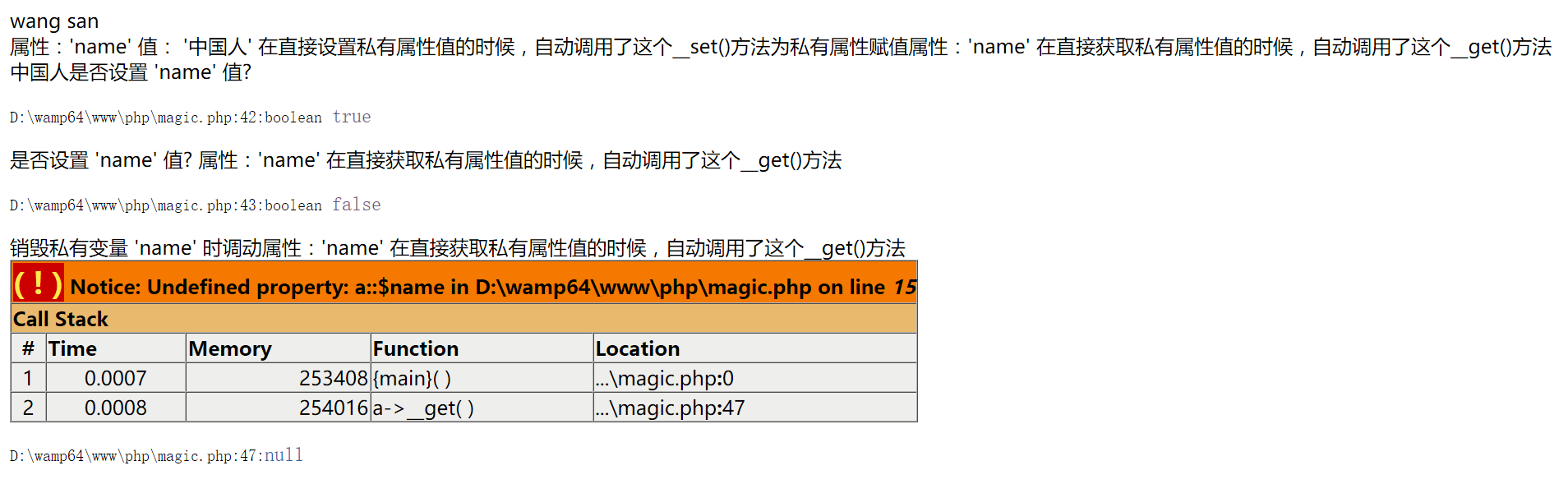# PHP常用的魔术方法及规则

1. __construct 具有构造函数的类会在每次创建新对象时先调用此方法;初始化工作执行。
2. __desstruct 对象的所有引用都被删除或者当对象被显式销毁时执行。
3.__call()在对象中调用一个不可访问方法时，__call() 会被调用。
4.__callStatic()在静态上下文中调用一个不可访问方法时，__callStatic() 会被调用。
5.__set() 在给不可访问的属性赋值时调用
6.__get() 读取不可访问的属性值是自动调用
7.__isset() 当对不可访问的私有属性使用isset或empty时自动调用
8.__unset() 当对不可访问的私有属性使用unset时；自动调用
9.__toString()当一个类的实例对象；被当成一个字符串输出时调用

<?php
class a
{

private $name = 'wang san'; //设置私有属性 public function __set($a,$val){ echo "属性：'$a' 值： '$val' 在直接设置私有属性值的时候，自动调用了这个__set()方法为私有属性赋值\n";$this->$a =$val;
}
//获取私有属性
public function __get($a){ echo "属性：'$a' 在直接获取私有属性值的时候，自动调用了这个__get()方法\n";
return $this->$a;
}
/**  当对不可访问属性调用 isset() 或 empty() 时，__isset() 会被调用。*/
public function __isset($name) { echo "是否设置 '$name' 值?\n";
return isset($this->$name);
}
/**  当对不可访问属性调用 unset()会被调用。*/
public function __unset($name) { echo "销毁私有变量 '$name' 时调动";
unset($this->$name);
}
/* 方法用于一个类被当成字符串时应怎样回应 */
public function __toString() {
return $this->name; } }$test = new a();

echo $test.'<br>'; // 输出：wang san __toString（）方法用于一个类被当成字符串时应怎样回应$test->name = "中国人";
echo $test->name; //输出 中国人 因为使用了魔术方法 __get 和 __set var_dump(isset($test->name)); //返回 true
var_dump(empty($test->name)); //返回 false unset($test->name); //销毁时；自动调用__unset

var_dump($test->name); //报错；未定义 Undefined property: a::$nameposted @ 2019-05-26 15:54  王默默  阅读(5181)  评论(0编辑  收藏  举报